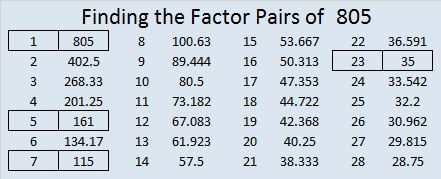# 805 and Level 4

23 × 35 = 805 so we shouldn’t be surprised that 805 is palindrome NN in BASE 34. N is the same as 23 in base 10. Thus NN can be derived from 23(34) + 23(1) = 23(34 + 1) = 23 × 35 = 805. NN obviously is divisible by 11 like all 2 digit palindromes are.

Since 23 = 22 + 1, should we expect that 805 is a palindrome in BASE 22? No, and that is for the same reason that not all multiples of 11 are palindromes.

Finding the factors in today’s puzzle shouldn’t be very difficult, but the last few might be trickier than the rest:Print the puzzles or type the solution on this excel file: 10-factors 801-806

• 805 is a composite number.
• Prime factorization: 805 = 5 x 7 x 23
• The exponents in the prime factorization are 1, 1, and 1. Adding one to each and multiplying we get (1 + 1)(1 + 1)(1 + 1) = 2 x 2 x 2 = 8. Therefore 805 has exactly 8 factors.
• Factors of 805: 1, 5, 7, 23, 35, 115, 161, 805
• Factor pairs: 805 = 1 x 805, 5 x 161, 7 x 115, or 23 x 35
• 805 has no square factors that allow its square root to be simplified. √805 ≈ 28.37252805 is the hypotenuse of a Pythagorean triple:

• 483-644-805, which is 3-4-5 times 161

805 can be written as the sum of three squares four ways:

• 25² + 12² + 6² = 805
• 24² + 15² + 2² = 805
• 20² + 18² + 9² = 805
• 18² + 16² + 15² = 805This site uses Akismet to reduce spam. Learn how your comment data is processed.